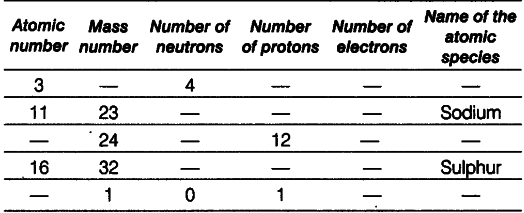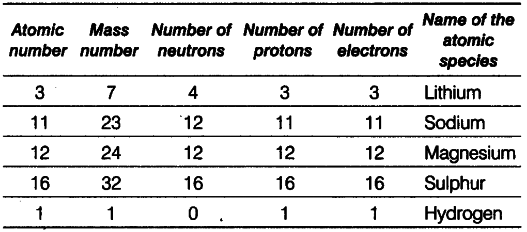# Complete the following table given below

Complete the following table.Explanation
(i) Lithium: Given, atomic number =’ 3 and number of neutrons = 4
Mass number - Atomic number + number of neutrons = 3 + 4 = 7 Number of protons = Atomic number
= Number of electrons = 3
(ii) Sodium : Given, atomic number =11 Number of protons = Number of electrons =11 Number of neutrons = Mass number -atomic number = 23-11 = 12
(iii) Magnesium Number of protons =12
Atomic number = Number of protons =12 Number of neutrons = Mass number-atomic number =24-12 = 12
(iv) SulphurnGiven, atomic number =16
Number of protons = Number of electrons =16 Number of neutrons = Mass number -atomic number = 32 —16 = 16
(v) Hydrogen Mass number = 1
Number of protons = 1
Number of neutrons = 0
Atomic number = Number of protons = 1 Number of electrons = Number of protons = 1 Because number of electrons is one, i.e. equal to that of protons, so the species is hydrogen atom.